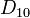# Difference between revisions of "Number of conjugacy classes in a subgroup may be more than in the whole group"

## Statement

It is possible to have a finite group$G$ and a subgroup$H$ of$G$ such that the Number of conjugacy classes (?) in$H$ is more than in$G$.

## Proof

### Example of the dihedral group of degree five

Further information: dihedral group:D10, cyclic group:Z5

The smallest pair of examples is where the group$G$ is$D_{10}$, the dihedral group of degree five and order ten, and$H$ is the subgroup is cyclic group:Z5. The group$D_{10}$ has four conjugacy classes: one of involutions, one identity element, and two conjugacy classes in the cyclic subgroup of order five. On the other hand,$H$ is an abelian group of order five hence has five conjugacy classes.

### Other dihedral examples

Further information: element structure of dihedral groups

More generally, for odd$n$, the dihedral group of order$2n$ and degree$n$ has$(n + 3)/2$ conjugacy classes, and the cyclic subgroup of order$n$ has$n$ conjugacy classes. For$n \ge 5$, the cyclic subgroup has more conjugacy classes than the whole group.

For even$n$, the dihedral group of order$2n$ has$(n + 6)/2$ conjugacy classes and the cyclic subgroup of order$n$ has$n$ conjugacy classes. For$n \ge 8$, the cyclic subgroup has more conjugacy classes than the whole group. The first example of this is cyclic group:Z8 in dihedral group:D16.